# MATCH Function In ExcelArticle byJeevan A Y## MATCH Function Definition

MATCH function in Excel returns the cell number of a specific value by looking it up in the given table array or range of cells. Thus, this function acts as a support function in lookup scenarios most of the time. This lookup value can be anything, from a cell reference to a number, text, or logical value.

For example, below is an Excel sheet listing students and their scores on the final exam. We can use the MATCH function here to fetch the relative position, i.e., row or column number of the lookup value ‘Sowmya,’ from the student list, wherever we want it on the sheet.

Here, we have put the lookup value ‘Sowmya” in cell D1. We will now enter the MATCH formula in any cell (D2 in this case) and use the reference cell D1 to locate the position of ‘Sowmya’ in the student list, i.e., A2:A9:

=MATCH(D1,A2:A9,0)

MATCH function here searches the position of the lookup value “Sowmya” in the range of cells A2:A9. It returns the result as 6, which is the actual position of “Sowmya” in the list.

Alternatively, we can put the lookup value (name, numeric, or text) in the MATCH function; instead of putting it in some cell and referencing it to get the result. See below:

=MATCH(“Sowmya”,A2:A9,0)

###### Key Takeaways
• The MATCH function is used in Excel to find the position of a specific value in the selected range of cells or table array.
• The formula often acts as a supporting function for other Excel Functions, like VLOOKUP.
• MATCH function always returns a numerical value as a result.
• The function can find the position of the lookup value even though the lookup value is not the same as in the lookup array by using wildcard characters.

### MATCH Function Syntax

Below is the formula for using the MATCH function in Excel:

MATCH function has just 3 parameters:

• Lookup_Value: The value whose position you want to locate in the lookup array (second argument). It is a mandatory argument.
• Lookup_Array: This will be either range or table array where you search for the lookup value. It is also a mandatory argument.
• [Match_Type]: It is an optional argument. This is the matching criteria that you need to define for the lookup value. It can be:

1 = It will look for the largest value, either less than or equal to the lookup_value you have provided and return the approximate match. It requires lookup_array to be arranged in the ascending order (lower to higher or A to Z).

0 = It will look for and return the exact match to the lookup_value, irrespective of how the data is arranged or sorted.

-1 = This will search for the smallest value, either greater than or equal to the lookup_value you have provided, and return the approximate match. It requires the lookup_array to be arranged in descending order (higher to lower or Z to A).

### How To Use?

Let us look at some of the basic examples to understand the usage of the MATCH function before jumping to advanced scenarios.

Below is the product list and the number of units sold for each product in a departmental store.

The steps to get the position for the product “Charger” using match function are as follows:

1. Enter the MATCH function in cell D1.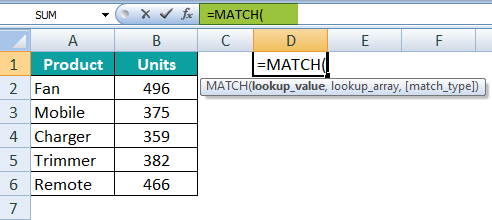2. We will now enter Lookup_Value, i.e., charger.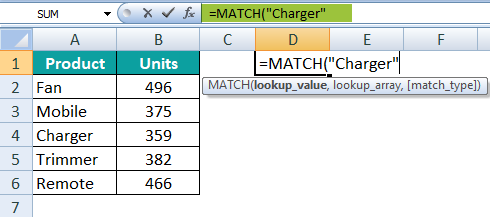3. We will then select the Lookup Array from range A2:A6, where we have a product list.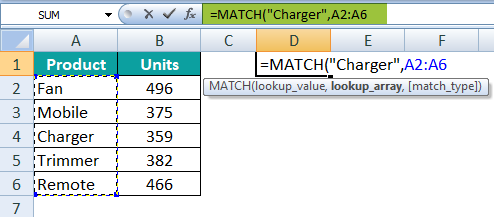4. The last argument of the MATCH function is to choose the matching type. We will be choosing 0 to find the exact match.This ends the construction of the formula. Finally, close the bracket and hit the “Enter” key to get the result.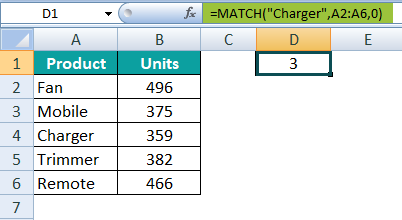The result of this function is 3, i.e., the position of “charger” in the lookup array A2:A6.

We need to keep in mind here that the counting of the position starts from the selected cell, not from the table’s header.

In the above example, we have supplied the lookup value directly to the formula, but we can also provide a cell reference for the lookup value. So, first, enter the lookup value in cell D1.

Now apply the same formula from above but this time for lookup value, select the cell reference D1.

This should return the same value as the above one. The advantage of this method is you can change the product name in cell D1, and cell D2 will automatically display the product’s position from the lookup array.

### Examples

The MATCH function supports other functions to perform complex calculations, such as VLOOKUP and INDEX functions. Let us look at some match function excel examples to understand it better:

#### Example #1: Partial Lookup Value in MATCH Function

Below is the list of a family with names and their age:

Here, the objective is to get the position of the name “Mirel Manchon,” but we have only the partial name as a lookup value in the reference cell D1.

To get the desired result, we need to use wildcard characters. As we have First Name here, we can combine the First Name with an asterisk (*) to match the remaining characters of the name.

It considers the first name, and then the wildcard asterisk (*) matches the remaining characters. This will locate the name Mirel Manchon in the list.

Step 1: Enter the MATCH formula in cell D2.

Step 2: Choose the reference cell D1 as the lookup value.

Step 3: We have only First Name as lookup value, so we need to combine the selected cell value with an asterisk (*), enter the ampersand (&) symbol, and an * in double-quotes.

Step 4: Next, we will enter lookup array A2:A10, where the actual name is present.

Step 5: The last argument will be match type. For this, we will enter 0 to get the exact match.

The result is 8.

Mirel Manchon’s position in the lookup array A2:A10 is 8. Therefore, even though we had only the First Name, the wildcard characters match the remaining characters of the name and give the exact result.

#### Example #2: MATCH Function with Different Match Types

We will look at how the MATCH function works with different match types. Below is the Excel sheet of various smartphone brands and their average price.

We will try to find the position of the brand Redmi in array A2:A5. So, we enter the match type as 0 to find the exact match.

The formula returns the result as 2 because the row number for the brand Redmi is 2 in the list.

Now let us find a numerical value with a different match type. For this, we need to sort the data in descending order.

For the average price of 19000, we will use 1 as the matching type to find the approximate match.

The formula returns the result as 1. This is because 19500 is greater than 18000 and lower than 20000. Both these prices do not exactly match the lookup value price of 19500. Hence, it returns a value lower than the lookup value.

For the same scenario, let us change the match type to -1 and see what happens.

This returns the #N/A error because the data is not sorted in the ascending order.

When we sort the data in ascending order, we will get the below result.

This returns the result as 2 in cell E4 because the lookup value 19500 is less than 20000 and higher than 18000. Hence, it returns the position of the nearest matching value.

#### Example #3: MATCH Function as Supportive Function

The MATCH function is often used as a supporting function for other functions. One such scenario will be to use it along with the VLOOKUP function.

Below is the attendance data for different employees across various months.

We need to find the attendance record for a particular employee named “Michael Kapser” (cell I2) across months from the above table.

Step 1: Enter the VLOOKUP function in cell J2.

Step 2: Enter lookup value, i.e., Michael Kapser as cell I2.

Make the selected cell I2 reference absolute (press the F4 key 3 times). Then, when you copy the formula to cells on the right side, this cell reference should remain the same for all the months.

Step 3: Next, we will enter the table array as A2:G10 or select the range of cells. We will make the reference cells absolute (press F4 once).

Step 4: Now, we will enter the column number from the selected range to get the lookup value for January, i.e., 2.

Entering column numbers for all the months is a difficult task. Hence, we will use the MATCH function to make it dynamic.

Enter the MATCH function now.

Step 5: Select the lookup value as January, i.e., cell J1.

Step 6: Select the lookup array as A1:G1 and make this reference absolute by pressing the F4 key once.

Step 7: The last argument is to select the exact match criteria. Enter 0 and close the bracket.

Step 8: This concludes the MATCH function. The last part of VLOOKUP is to select the matching type, i.e., enter 0, which will be the exact match.

Close the bracket and hit the “Enter” key to get the attendance days for Michael Kapser for January.

The MATCH function will return the result as 29. Now copy the formula to the right side of the cells to get the result for all the months.

In this way, we can give the column numbers to the VLOOKUP dynamically by using the MATCH function.

### Important Things To Note

• MATCH function gives only the numerical value as an output.
• It can search for any lookup value – a cell reference, number, text, or logical value.
• If the entered lookup value is not found in the lookup array, it will return the #N/A error.
• The MATCH function returns a value based on the match type we select – 0 will be the exact match, -1 will be greater than or equal to the lookup value, and 1 will be less than or equal to the lookup value. The last two match types will be approximate matches.

### Frequently Asked Questions (FAQs)

What does the MATCH function do in excel?

MATCH function will return the number for the lookup value by searching it in the lookup array. The function works for both horizontal and vertical datasets. Irrespective of how the data set is sorted, the MATCH function will return the position of the lookup value in the selected array.

An Excel sheet listing students and their final exam scores, for example, can be found below. We may use the MATCH function to get the relative location of the lookup value “Sowmya” from the student list, i.e., the row or column number, wherever we want it on the sheet, using the MATCH function.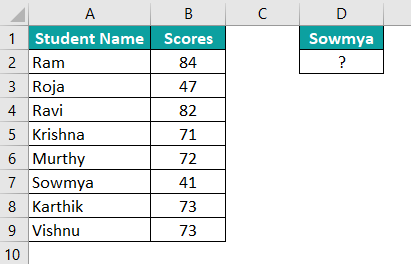In cell D1, we have entered the lookup value “Sowmya.” We’ll now enter the MATCH formula in any cell (in this case, D2) and utilize the reference cell D1 to find ‘Sowmya’ in the student list, i.e., A2:A9:

=MATCH(D1,A2:A9,0)

In this case, the MATCH function looks for the lookup value “Sowmya” in the range of cells A2:A9. So, it gives you the number 6, which is the position of “Sowmya” in the list.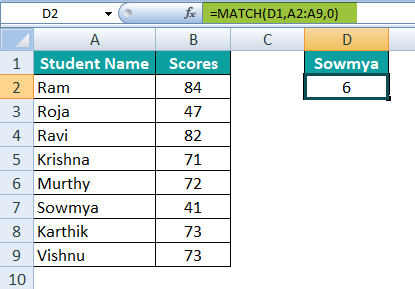Why do we need to select 0 – exact match as the last argument in the MATCH function?

Entering 0 as the last argument in the MATCH function gives the user the exact match for the lookup value. So, if no exact match is found, the function will return the #N/A error.

How to MATCH function in VLOOKUP?

When looking up a value in the table array for more than one column, we need to manually enter the column number to the VLOOKUP. But by using the MATCH function, we can fetch the column number dynamically with the same header as we have in the original table.